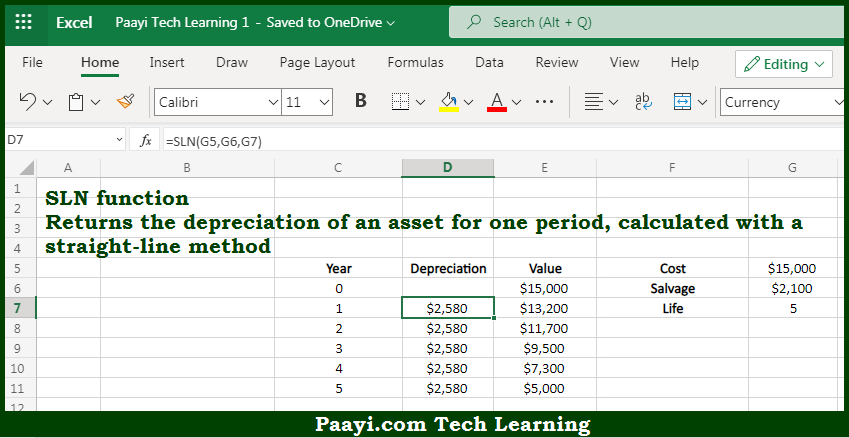# Learn How to Use Microsoft Excel SLN Function

Written by | 0 Comments | 546 Views

In this article, you will learn how to use the Microsoft Excel SLN function and its prime function in Microsoft Excel. You will also get to know the Microsoft Excel SLN function return value and syntax with the help of some examples.

Microsoft Excel SLN Function

The main purpose of the Microsoft Excel SLN function is to get the depreciation at the straight line. That implies, with the help of the SLN function you can able to return the depreciation of an asset for one period, calculated with a straight-line method. It should be noted that the calculated depreciation is based on initial asset cost, salvage value, and the number of periods over which the asset is depreciated. So, with the help of the SLN function, you can able to get the depreciation at the straight line.

Return Value of SLN Function

The return value will be the depreciation in the given period.

Syntax of SLN Function

=SLN(cost, salvage, life)

Where the arguments:

• cost: This is the initial cost of the asset.
• salvage: This is the asset value at the end of depreciation.
• life: This is the period over which the asset is depreciated.

How to Use Microsoft Excel SLN Function?So we know that Microsoft Excel SLN function you can able to get the depreciation at the straight line. That implies, with the help of the SLN function you can able to return the depreciation of an asset for one period, calculated with a straight-line method. It should be noted that the calculated depreciation is based on initial asset cost, salvage value, and the number of periods over which the asset is depreciated. So, with the help of the SLN function, you can able to get the depreciation at the straight line.